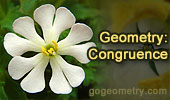# Online Geometry: Congruence, Rotation, Translation, Reflection - Page 9Proposed Problem 23
Right Triangle, altitude, incircles and inradii.

Proposed problem 19
Right Triangle and Excenter

Proposed Problem 17.
Right triangle, altitude, and angles.

Proposed Problem 16.
Triangle, cevian, perpendicular, and angles.

Proposed Problem 15.
Triangle, cevian, sum of segments, and angles.

Proposed Problem 14.
Triangle, median, and angles.

Proposed Problem 13. Triangle, cevian, equal segments, and angles.

Proposed Problem 12.
Triangle, cevian, equal segments, and angles.

Proposed Problem 11.
Right triangle, cevian, and angles.

Proposed Problem 10.
Triangle, cevian, equal segments, and angles.

Previous | 1 | 2 | 3 | 4 | 5 | 6 | 7 | 8 | 9 | 10 | Next

 Home | Geometry | Problems | Email | By Antonio Gutierrez